# The Problem of Chunky Data

Thus, for subgroups of size n = 3 or larger, the measurement increment borders on being too large when there are only five possible values within the limits on the range chart. Fewer values within are indicative of chunky data.

As may be seen in figure 3, the running records of the averages are trying to tell us the same story. However, the fewer possible values in figure 2 make the running record look more “chunky” than that in figure 1. This chunkiness also results in highs and lows that are more extreme in figure 2. At the same time, the similarity of rounded values within each of the subgroups results in many zero ranges in figure 2. These deflate the average range, which in turn deflates the limits.

Thanks,
Quality Digest

منبع: https://www.qualitydigest.com/inside/statistics-article/problem-chunky-data-071023.html

When the measurement increments used are too large for the job, the limits on a process behavior chart, as well as other statistical techniques, can be distorted. This distortion can lead to spurious results. Fortunately, this problem is easily detected by ordinary, production-line process behavior charts. No special studies are necessary; no standard parts or batches are needed. You simply need to recognize the telltale signs.

Since the problem with chunky data comes from the inability to detect variation within the subgroups, the solution consists of increasing the ability of the measurements to detect that variation.

Our PROMISE: Quality Digest only displays static ads that never overlay or cover up content. They never get in your way. They are there for you to read, or not.

However, someone has to pay for this content. And that’s where advertising comes in. Most people consider ads a nuisance, but they do serve a useful function besides allowing media companies to stay afloat. They keep you aware of new products and services relevant to your industry. All ads in Quality Digest apply directly to products and services that most of our readers need. You won’t see automobile or health supplement ads.

While the limits on the average chart change slightly, the false alarms persist. Using a standard deviation chart won’t remedy the problem of chunky data.

To illustrate the effect of using a measurement increment that is too large, we shall round off the measurements in figure 1 to the nearest hundredth of an inch. While we would never do this in practice, we do it here to simulate what would happen if the measurements had only been recorded to two decimal places. After rounding these data, the averages and ranges were recomputed and a new average and range chart was obtained. In figure 2 we find four averages and two ranges outside the limits. The usual interpretation of the chart in figure 2 would be that these data show a lack of homogeneity, and that the underlying process is changing in some manner.Figure 7: The basis for detecting chunky data with a range chart

Statistics

## The Problem of Chunky Data

### What happens when the measurement increment gets too large?

Here, except for n = 2, round-off begins to introduce bias as soon as SD(X) gets smaller than twice the measurement increment. As before, these curves all plunge on the left, and the limits shrink to oblivion as SD(X) gets smaller and smaller relative to the measurement increment.

Therefore, the procedure to check for chunky data consists of three steps:
1. Determine the measurement increment used. This is done by inspecting either the ranges or the original data.
2. Determine the upper and lower limits for the range chart. This is done in the usual manner.
3. Determine how many possible values for the range fall within the range limits and apply the rules given above.

### Fixing chunky data

One solution is to use smaller measurement increments. If you have been round­ing your measurements too aggressively, you can solve the problem of chunky data by sim­ply recording an additional digit for each measurement. Even if there is some uncertainty in that extra digit, its inclusion can actually improve the quality of your data. So, regardless of tradition, if your data are chunky because you have been rounding off your measurements, you need to start recording extra digits.

The ranges will always have the same increments as the original data. Thus, the ranges in figure 1 are all multiples of one-thousandth of an inch. The range chart from figure 1 is reproduced in figure 4. The upper range limit is 0.0181. Dividing by 0.001, we find 19 possible values (from 0 to 18 thousandths) within the limits on the range chart.

The data in figure 1 are the measurements of a physical dimension on a plastic knob. These data were recorded to the nearest one-thousandth of an inch (0.001 in.). There are no signals of exceptional variation on either the average chart or the range chart. Since these data show no evidence of a lack of homogeneity, we would conclude that the process producing these rheostat knobs is being operated predictably.

Quality Digest does not charge readers for its content. We believe that industry news is important for you to do your job, and Quality Digest supports businesses of all types.

On the right, all the curves are on the line marked 0% bias, and the formulas will have an average bias of zero. As we move to the left, the standard deviation shrinks and the curves deviate from the unbiased line.

The range chart from figure 2 is given in figure 5. There, the upper range limit is 0.0102. Dividing by 0.01, we find only two possible values (0 and 1 hundredth) within the limits on the range chart.

However, we know that the charts in figure 1 and those in figure 2 both represent the same process. The only difference between the two charts is the measurement increment used. Based on figure 1, we have to conclude that the “signals” in figure 2 are actually false alarms created by the round-off operation.

Data analysis consists of filtering out the noise so we can detect any signals that may be present. Process behavior charts use three-sigma limits to filter out the “noise” of routine variation. The formulas for these limits are based on “unbiased estimators.” The mathematical support for these unbiased estimators rests upon an assumption that the data come from a continuum of values. As long as the standard deviation for the data is larger than the measurement increment, this assumption is reasonable and the formulas will work as advertised. But as the data become more discrete, the formulas will become increasingly biased.

Figure 6 shows the average bias introduced by round-off into the formulas based on the average range. The average bias is shown on the vertical scale, while the horizontal scale shows the size of the standard deviation of the product measurements, SD(X), relative to the measurement increment.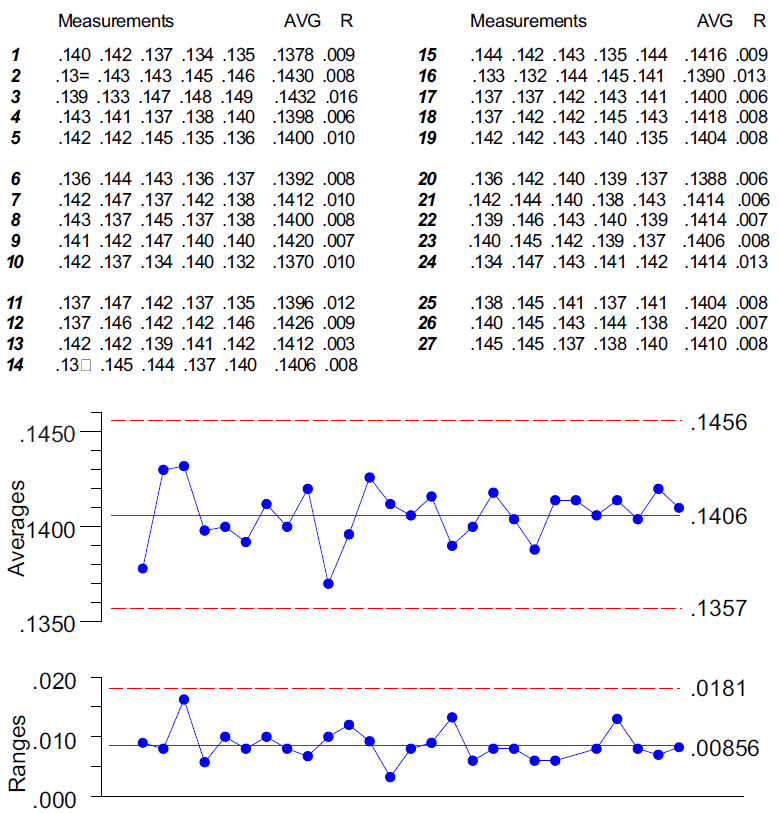Figure 1: Average and range chart when rheostat knob data are recorded to 0.001 inch

Another solution to the problem of chunky data is to increase the variation within the subgroups. This will increase the ability of your current measurement system to detect varia­tion within the subgroups. With an average chart, this will usually involve a change in what a subgroup represents. When a single sub­group represents several successive parts coming off a line, you can usually increase the variation sufficiently by simply expanding the subgroup by waiting between sampled parts so each subgroup represents a longer period of time. When the within-subgroup variation becomes detectable, the visible effects of chunky data on the average and range chart will disappear.

The problem seen in figure 2 is due to the inability of the measurement increments to properly reflect the process variation. When these measurements are rounded to the nearest hundredth of an inch, most of the information about variation is lost in the round-off. As a result, the rounded data have many zero ranges, even though the original data have no zero ranges. These zero ranges deflate the average range and tighten the computed limits. At the same time, the greater discreteness for both the averages and the ranges will prevent the running records from shrinking with the limits. Eventually it becomes inevitable that some points will fall outside the artificially tightened limits even though the process itself is predictable.

If we define the borderline safe condition to be that point at which the standard deviation of the product measurements, SD(X), is equal to the measurement increment, then the limits for the range chart will have the following form:

Published: Monday, July 10, 2023 – 12:03

So while the highs and lows get emphasized by the larger measurement increments, the limits get squeezed. When this happens it’s inevitable that the running record and limits will eventually collide and produce false alarms.

Before we can say that our average and standard deviation chart is likely to be free from the biases introduced by chunky data, we will want to have an average standard deviation statistic that is greater than twice the measurement increment.

Figures 6 and 9 show that the effects of chunky data are eventually the same regard­less of whether we’re using subgroup ranges or subgroup standard deviations. Once SD(X) is less than one-half measurement increment, the limits will plummet toward zero regardless of subgroup size and regardless of which measure of dispersion we use.

### Summary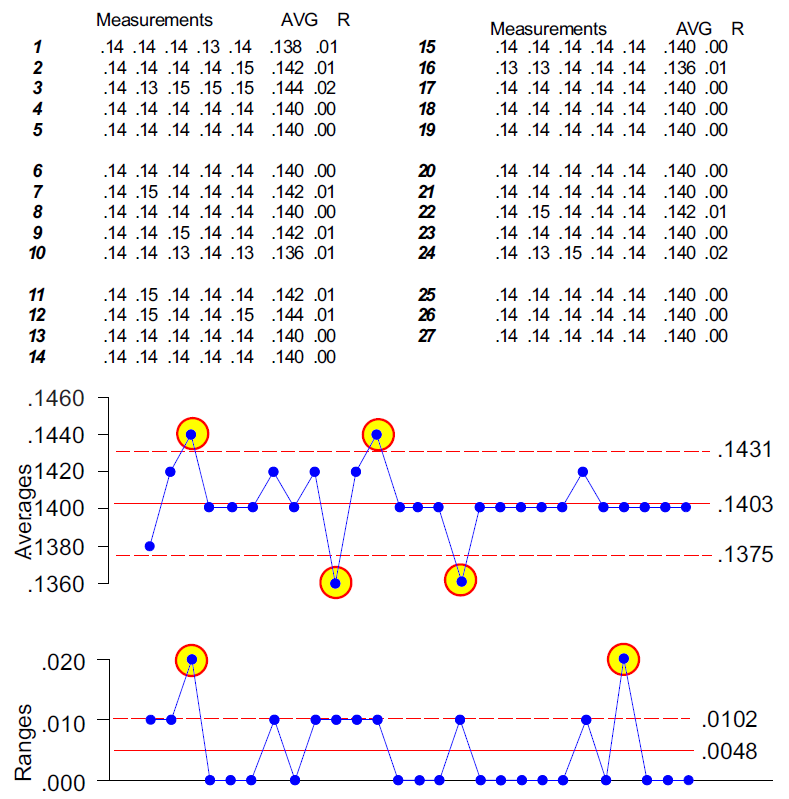Figure 2: Average and range chart when rheostat knob data are rounded to 0.01 inch

The bottom curve in figure 6 is for the average of two-point moving ranges. The remaining curves, in ascending order according to their left-hand end points, are for average ranges based on subgroups of size 2, 3, 4, 5, 6, 8, and 10.

On the right side of figure 6, we see that the formulas based on the average range will remain unbiased as long as SD(X) is larger than two-thirds of the measurement increment.

If the current measurement system will not provide you with additional digits for your obser­vations, then you may need to consider changing the measurement system.

Data are said to be chunky when the distance between the possible values becomes too large. For example, what would happen if measurements of the heights of different individ­uals were made to the nearest yard? Clearly, the variation from person to person would be lost in the round-off, and any attempt to characterize the variation in heights would be flawed. When the round-off of the measurements begins to obliterate the variation within the data, you will have chunky data. The effect that chunky data have on process behavior charts is illustrated by the following example.Figure 6: The average bias for formulas using the average range

These values are tabled for subgroup sizes of n = 2 to n = 10 in figure 7. Consideration of these limits reveals the number of possible values within the limits on a range chart at this borderline safe condition.

For subgroups of size n = 2, the measurement increment borders on being too large when there are only four possible values within the limits on the range chart. Fewer values within are indicative of chunky data.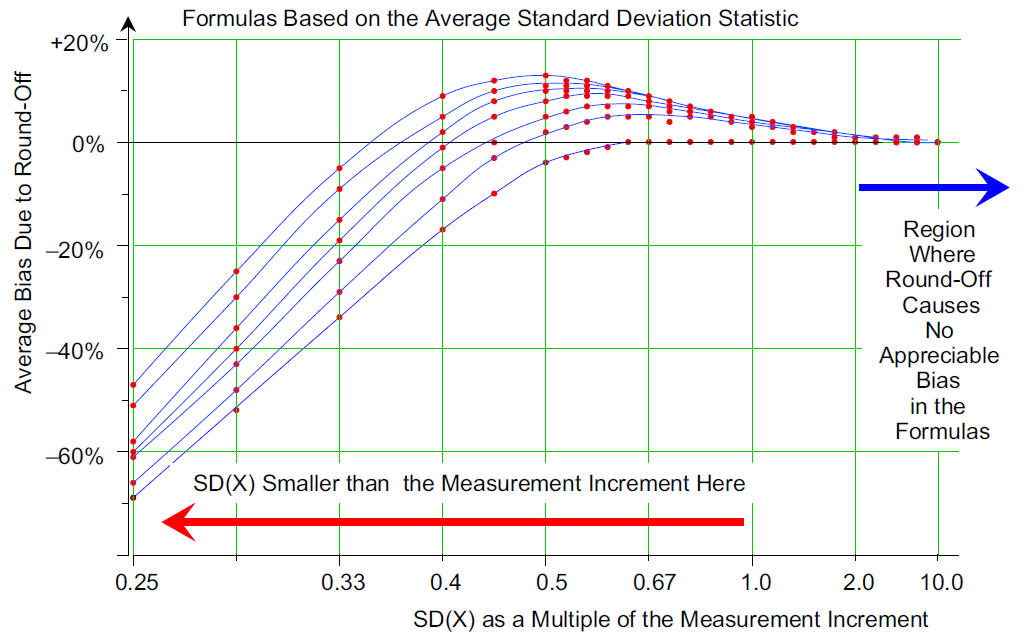Figure 9: The average bias for formulas using the average standard deviation

For XmR charts and average charts with subgroups of size n = 2, you need to have at least four possible values for the range below the upper range limit to be safe from the effects of chunky data.

So how can we spot this problem? The very look of the running records is one clue; the abundance of zero ranges is another. However, the clear-cut, unequivocal indicator of chunky data is the number of possible values within the limits on the range chart.

Most problems with process behavior charts are fail-safe. That is, the charts will err in the direction of hiding a signal rather than causing a false alarm. Because of this feature, when you get a signal, you can trust the chart to guide you in the right direction. Chunky data are one of two exceptions to this fail-safe feature of the process behavior chart. (The other exception was the topic of last month’s column.)

By comparing figures 1 and 2, it should be apparent that chunky data can make a predictable process appear to be unpredictable.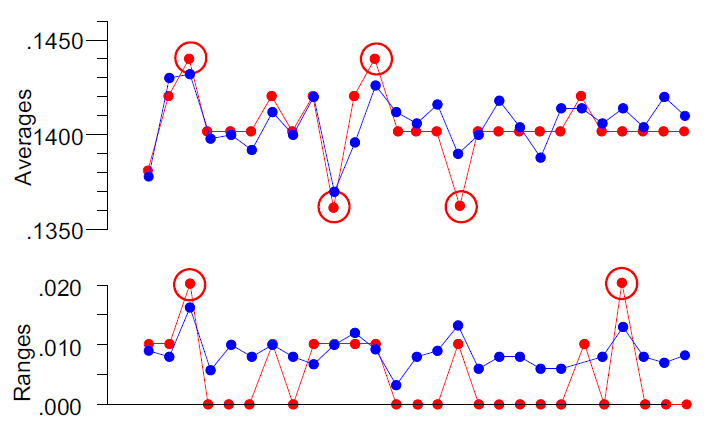Figure 3: Running records from figures 1 and 2

When you fail to meet one of these minimums, your data are chunky, and the limits may be deflated by the round-off inherent in the measurement increment you are using.Figure 5: Range chart from figure 2

When SD(X) gets smaller than this, the round-off will begin to introduce biases into the formulas. While the top four curves show a region with some positive biases, ultimately all the curves plunge on the left, and the limits will shrink to oblivion as SD(X) gets smaller relative to the measurement increment.

### The borderline conditions

Figure 1, with 19 possible values, shows no problem due to chunky data. Figure 2, with only two possible values, shows data that are definitely chunky—the measurement increment is too large for the purposes of creating a useful and meaningful process behavior chart. Since chunky data can create false alarms, you can’t safely interpret the “signals” of figure 2 as evidence of exceptional process variation. Thus, while chunky data may still be used for inspection, they can’t be used to characterize process behavior, and neither should they be used with any other statistical inference technique.

While these detection rules will work with range charts and moving range charts, they will not work with other charts for dispersion. This is because the range is the only measure of dispersion that preserves the discreteness of the original measure­ments.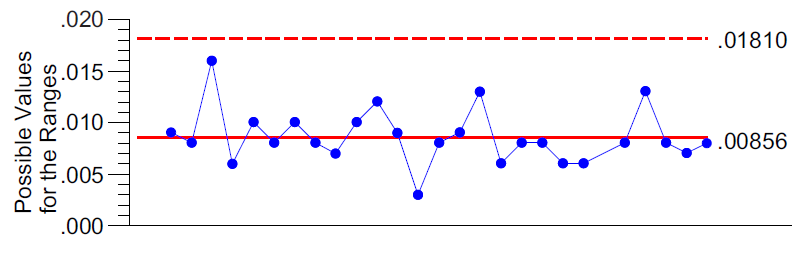Figure 4: Range chart from figure 1

Lower range limit = D3 MEAN(R) = D3 d2 SD(X) = D3 d2 measurement increments

Can we remedy the problem of chunky data by using subgroup standard deviations in place of the ranges? No, we can’t. Figure 8 shows the average and standard deviation chart for the data of figure 2.

Upper range limit = D4 MEAN(R) = D4 d2 SD(X) = D4 d2 measurement increments

So, how many values inside the limits do you need to be safe from the effects of chunky data?

### Detecting chunky data

The solution to chunky data requires less round-off in the measurements or an increase in the variation within the subgroups. Otherwise, your process behavior charts are likely to be misleading.

منتشر شده در
دسته‌بندی شده در اخبار